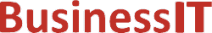O nás     Inzerce     KontaktSpolehlivé informace o IT již od roku 2011[Linux manuál]

# log, logf, logl: přirozená logaritmická funkce

Originální popis anglicky: log, logf, logl - natural logarithm function

Návod, kniha: POSIX Programmer's Manual

## STRUČNĚ

#include <math.h>

double log(double x);

float logf(float x);

long double logl(long double x);

## POPIS / INSTRUKCE

These functions shall compute the natural logarithm of their argument x, log_ e(x).
An application wishing to check for error situations should set errno to zero and call feclearexcept(FE_ALL_EXCEPT) before calling these functions. On return, if errno is non-zero or fetestexcept(FE_INVALID | FE_DIVBYZERO | FE_OVERFLOW | FE_UNDERFLOW) is non-zero, an error has occurred.

## NÁVRATOVÁ HODNOTA

Upon successful completion, these functions shall return the natural logarithm of x.
If x is ±0, a pole error shall occur and log(), logf(), and logl() shall return -HUGE_VAL, -HUGE_VALF, and -HUGE_VALL, respectively.
For finite values of x that are less than 0,  or if x is -Inf, a domain error shall occur, and  either a NaN (if supported), or  an implementation-defined value shall be returned.
If x is NaN, a NaN shall be returned.
If x is 1, +0 shall be returned.
If x is +Inf, x shall be returned.

## CHYBY / ERRORY

These functions shall fail if:
Domain Error
The finite value of x is negative,  or x is -Inf.
If the integer expression (math_errhandling & MATH_ERRNO) is non-zero, then errno shall be set to [EDOM]. If the integer expression (math_errhandling & MATH_ERREXCEPT) is non-zero, then the invalid floating-point exception shall be raised.
Pole Error
The value of x is zero.
If the integer expression (math_errhandling & MATH_ERRNO) is non-zero, then errno shall be set to [ERANGE]. If the integer expression (math_errhandling & MATH_ERREXCEPT) is non-zero, then the divide-by-zero floating-point exception shall be raised.

The following sections are informative.

None.

## APPLICATION USAGE

On error, the expressions (math_errhandling & MATH_ERRNO) and (math_errhandling & MATH_ERREXCEPT) are independent of each other, but at least one of them must be non-zero.

None.

None.

## SOUVISEJÍCÍ

exp() , feclearexcept() , fetestexcept() , isnan() , log10() , log1p() , the Base Definitions volume of IEEE Std 1003.1-2001, Section 4.18, Treatment of Error Conditions for Mathematical Functions, <math.h> Portions of this text are reprinted and reproduced in electronic form from IEEE Std 1003.1, 2003 Edition, Standard for Information Technology -- Portable Operating System Interface (POSIX), The Open Group Base Specifications Issue 6, Copyright (C) 2001-2003 by the Institute of Electrical and Electronics Engineers, Inc and The Open Group. In the event of any discrepancy between this version and the original IEEE and The Open Group Standard, the original IEEE and The Open Group Standard is the referee document. The original Standard can be obtained online at http://www.opengroup.org/unix/online.html .
 2003 IEEE/The Open Group
©2011-2020 BusinessIT.cz, ISSN 1805-0522 | Názvy použité v textech mohou být ochrannými známkami příslušných vlastníků.
Provozovatel: Bispiral, s.r.o., kontakt: BusinessIT(at)Bispiral.com | Inzerce: Best Online Media, s.r.o., zuzana@online-media.cz
O vydavateli | Pravidla webu BusinessIT.cz a ochrana soukromí | pg(9310)